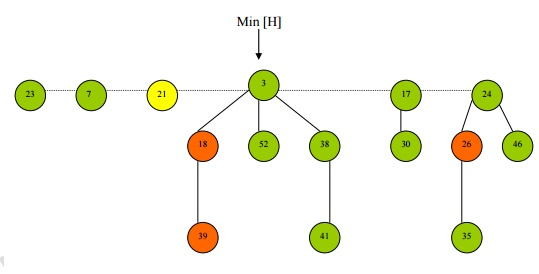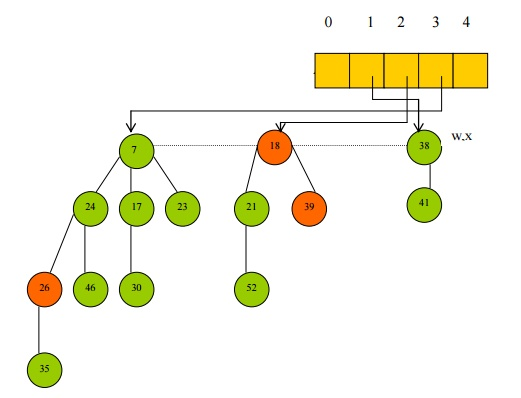# Fibonacci Heaps

A binomial tree of order 0 is a single node. A binomial tree of order k has a root of degree k and its children are roots of binomial trees of orders k-1, k-2, ..., 2, 1, 0 (in order).

FIBONACCI HEAPS:

Binomial Tree:

A binomial tree of order 0 is a single node. A binomial tree of order k has a root of degree k and its children are roots of binomial trees of orders k-1, k-2, ..., 2, 1, 0 (in order). A binomial tree of order k has 2k nodes.Data Structures:

Â·        Heap ordered Trees

Â·   Rooted, but unordered

Â·        Children of a node are linked together in a Circular, doubly linked List, E.g node 52Node Pointers

-  left [x]

-  right [x]

-  degree [x]

-  number of children in the child list of x

-  mark [x]

Properties:

Â·        Collection of unordered Binomial Trees.

Â·        Support Mergeable heap operations such as Insert, Minimum, Extract Min, and Union in constant time O(1)

Â·        Desirable when the number of Extract Min and Delete operations is small relative to the number of other operations.

Â·        Most asymptotically fastest algorithms for computing minimum spanning trees and finding single source shortest paths make use of the Fibonacci heaps.

Fibonacci Heap Operations:

Make Fibonacci Heap

Â·        Assign N[H] = 0, min[H] = nil

Â·        Amortized cost is O(1)

Find Min

Â·        Access Min[H] in O(1) time

Uniting 2 Fibonacci Heaps

Â·        Concatenate the root lists of Heap1 and Heap2

Â·        Set the minimum node of the new Heap

Â·        Free the 2 heap objects

Â·        Amortized cost is equal to actual cost of O(1)

Insert

Â·        Amortized cost is O(1)

Â·        Initialize the structural fields of node x, add it to the root list of H

Â·        Update the pointer to the minimum node of H, min[H]

Â·        Increment the total number of nodes in the Heap, n[H]

Example:Â·   Node 21 has been inserted

Â·        Red Nodes are marked nodes â€“ they will become relevant only in the delete operation.Extract Minimum Node

Â·        Amortized cost is O(D(n))

Â·        Make a root out of each of the minimum nodeâ€źs children.

Â·        Remove the minimum node from the root list, min ptr to right(x)

Â·        Consolidate the root list by linking roots of equal degree and key[x] <= key[y], until every root in the root list has a distinct degree value. (uses auxiliary array)Â·        Root Nodes are stored in the auxiliary array in the index corresponding to their degree.

Â·        Hence node 7 is stored in index 3 and node 21 is stored in index 0.Â·        Further, node 18 is stored in index 1

Â·        There are no root nodes of index 2, so that array entry remains empty

Â·        Now the algorithm iterates through the auxiliary array and links roots of equal degree such that key[x] <= key[y],Â·        Nodes 21 and 52 are linked so that node 21 has degree 1, and it is then linked to node 18Â·        The pointers of the auxiliary array are updated.

Â·        But there are now no more remaining root nodes of duplicate degrees.Â·   Extract-Min is now done.

Â·        The pointer min[H] is finally updated to the new minimum.A node is marked if:

Â·        At some point it was a root then it was linked to anther node and 1 child of it has been cut.

Â·        Newly created nodes are always unmarked

Â·        A node becomes unmarked whenever it becomes the child of another node.

Decrease-Key

Â·        Amortized cost is O(1).

Â·        Check that new key is not greater than current key, then assign new key to x

Â·        If x is a root or if heap property is maintained, no structural changes

Â·        Else

o  Cut x: make x a root, remove link from parent y, clear marked field of x

o   Perform a Cascading cut on xâ€źs parent y (relevant if parent is marked):

Â§    if unmarked: mark y, return

Â§    if marked: Cut y, recurse on node yâ€źs parent

Â§    if y is a root, return

Â·        The Cascading cut procedure recurses its way up the tree until a root, or an unmarked node is found.

Â·        Update min[H] if necessary.

Example:

Â·        Suppose we want to decrease the keys of node 46  to 15.Â·        Since the new key of node 46 is 15 it violates the min-heap property, so it is cut and put on the root.

Â·        Suppose now we want to decrease the key of node 35 to 5.Â·        So node 5 is cut and place on the root list because again the heap property is violated.

Â·        But the parent of node 35, which is node 26, is marked, so we have to perform aÂ·        The cascading cut involves cutting the marked node, 26, unmarking it, and putting it on the root list.

Â·        We now repeat this procedure (recurse) on the marked nodes parent until we hit a node on the root list.Â·        Since the next node encountered in the cascading cut procedure is a root node â€“ node 7 â€“ the procedure terminates.

Â·        Now the min heap pointer is updated.Delete-Key

Â·        Amortized cost is O(D(n))

Â·        Call Decease-Key on the node x that needs to be deleted in H, assigning negative infinity to it.

Â·        Call Extract-Min on H.

Study Material, Lecturing Notes, Assignment, Reference, Wiki description explanation, brief detail
Programming and Data structures : Advanced Non-Linear Data Structures : Fibonacci Heaps |